# Selina Solutions Concise Maths Class 7 Chapter 4: Decimal Fractions (Decimals) Exercise 4D

Selina Solutions Concise Maths Class 7 Chapter 4 Decimal Fractions (Decimals) Exercise 4D mainly discusses the concept of terminating, non terminating and recurring decimals. The method of converting them and the steps to be followed are explained in simple language for a better understanding among students. Students can improve their time management skills by solving problems using the solutions designed by faculty at BYJU’S. Selina Solutions Concise Maths Class 7 Chapter 4 Decimal Fractions (Decimals) Exercise 4D, PDF links are available below.

## Selina Solutions Concise Maths Class 7 Chapter 4: Decimal Fractions (Decimals) Exercise 4D Download PDF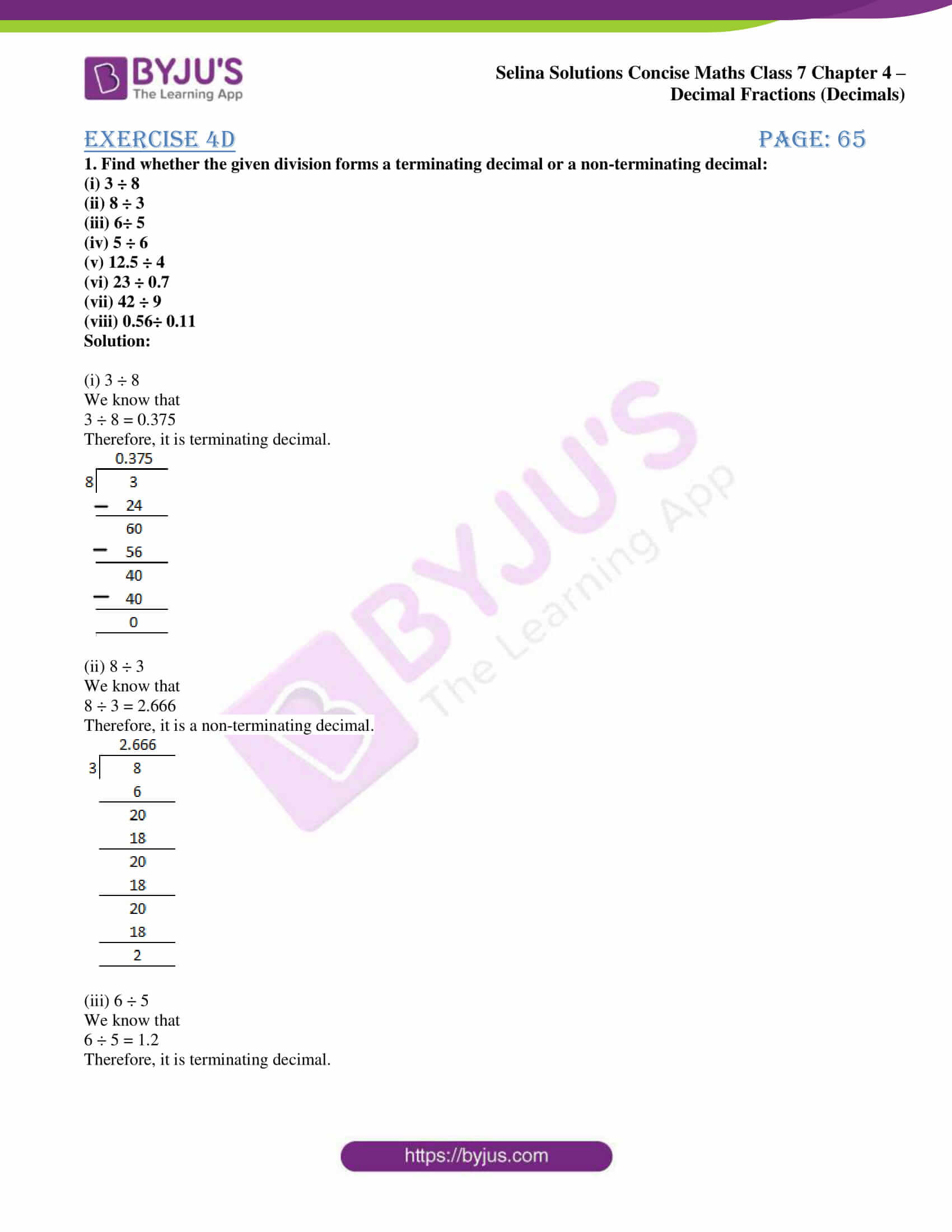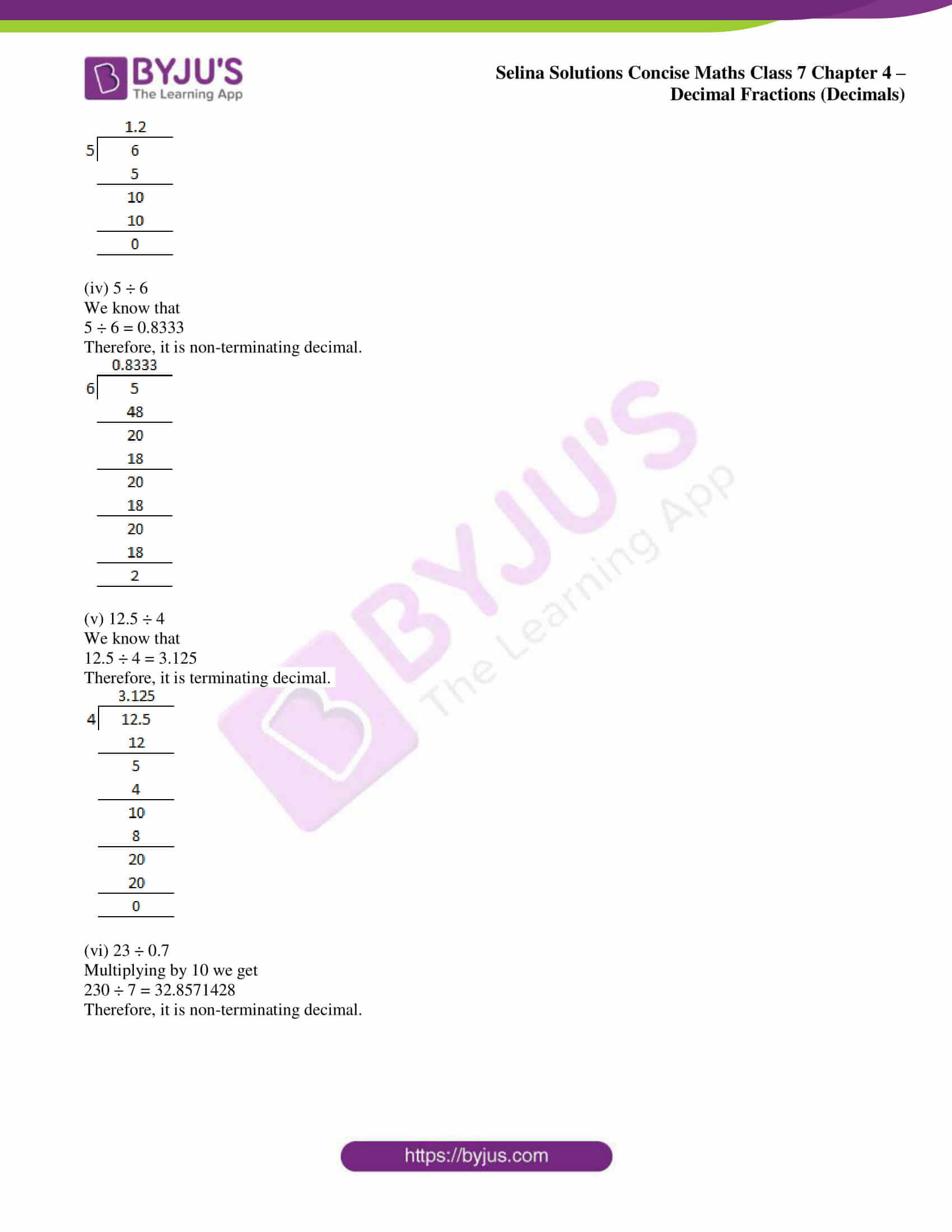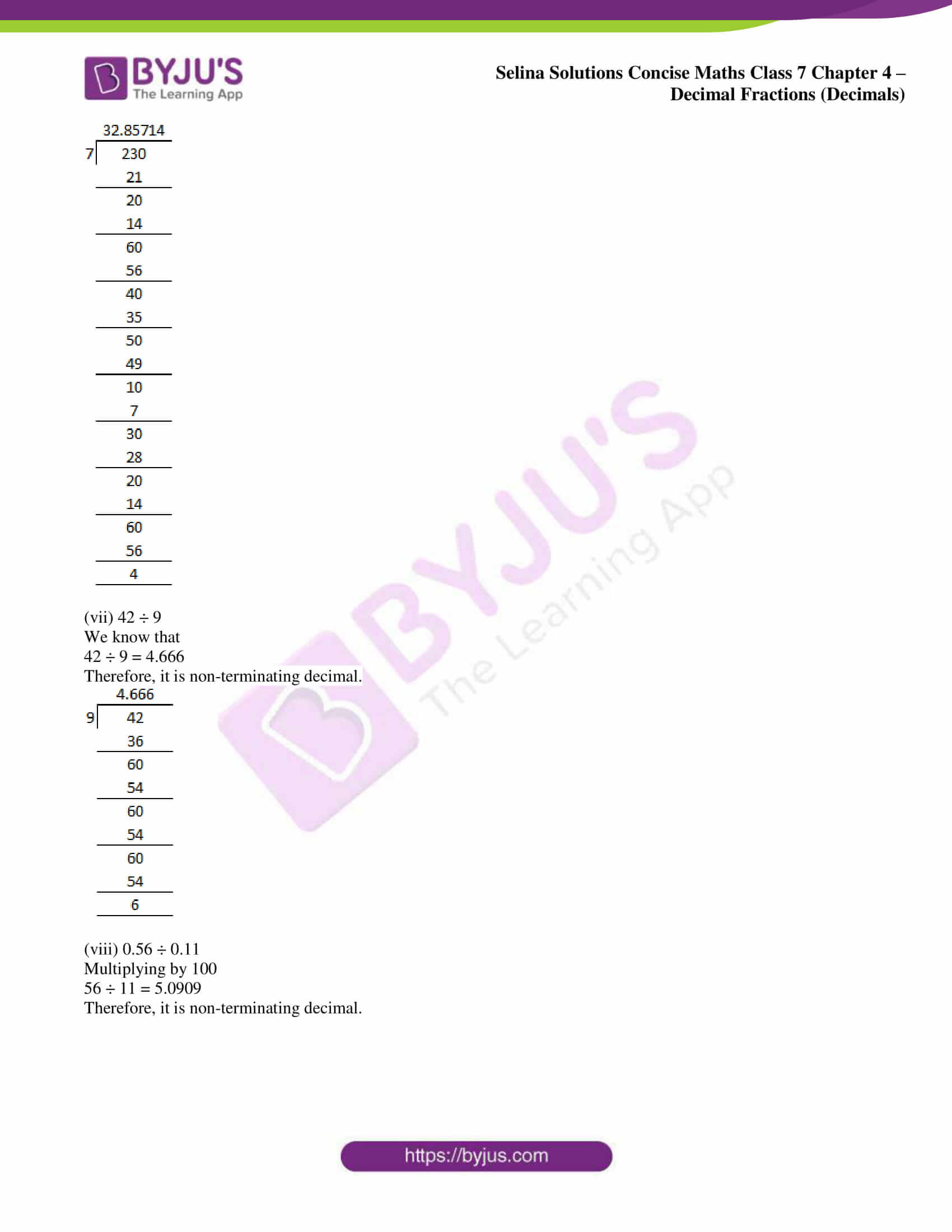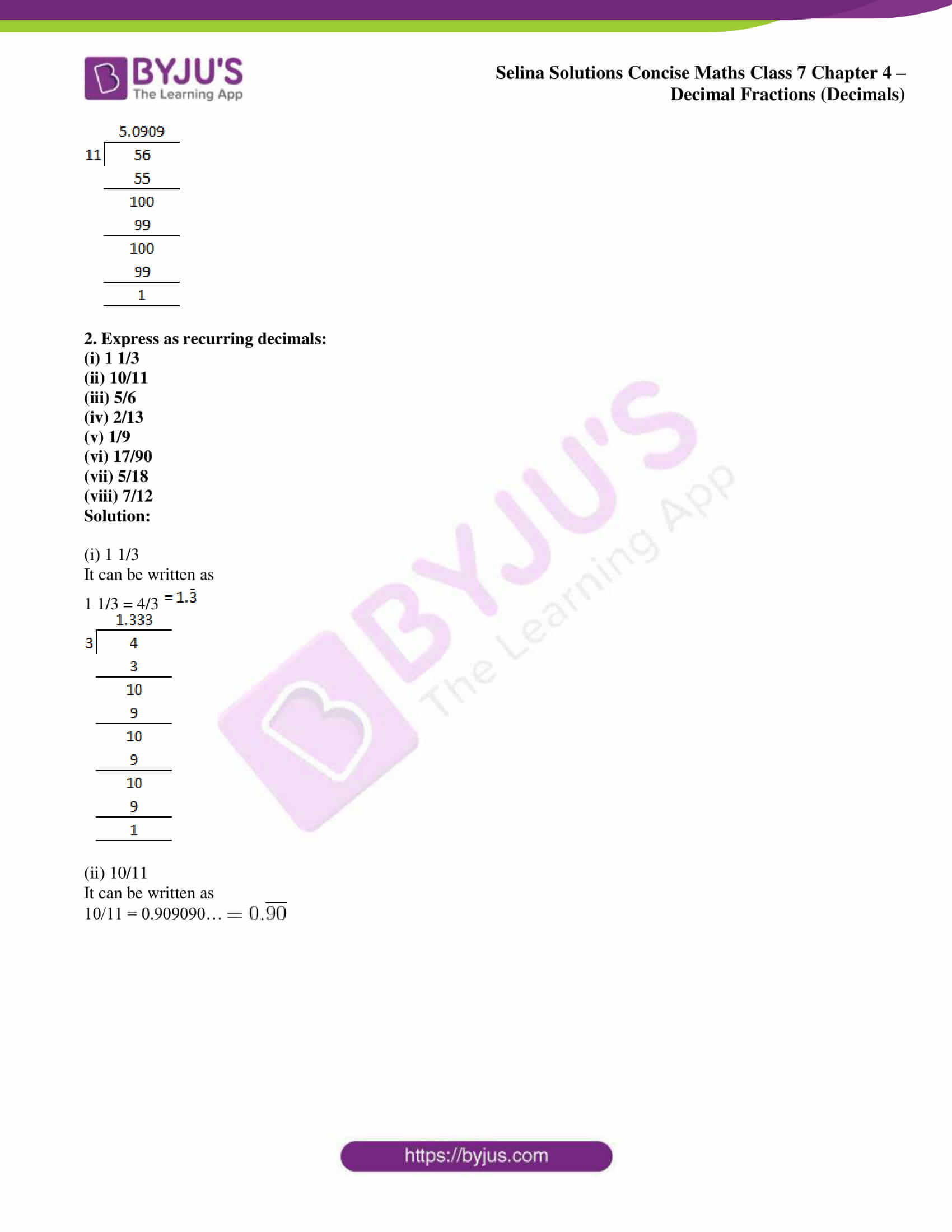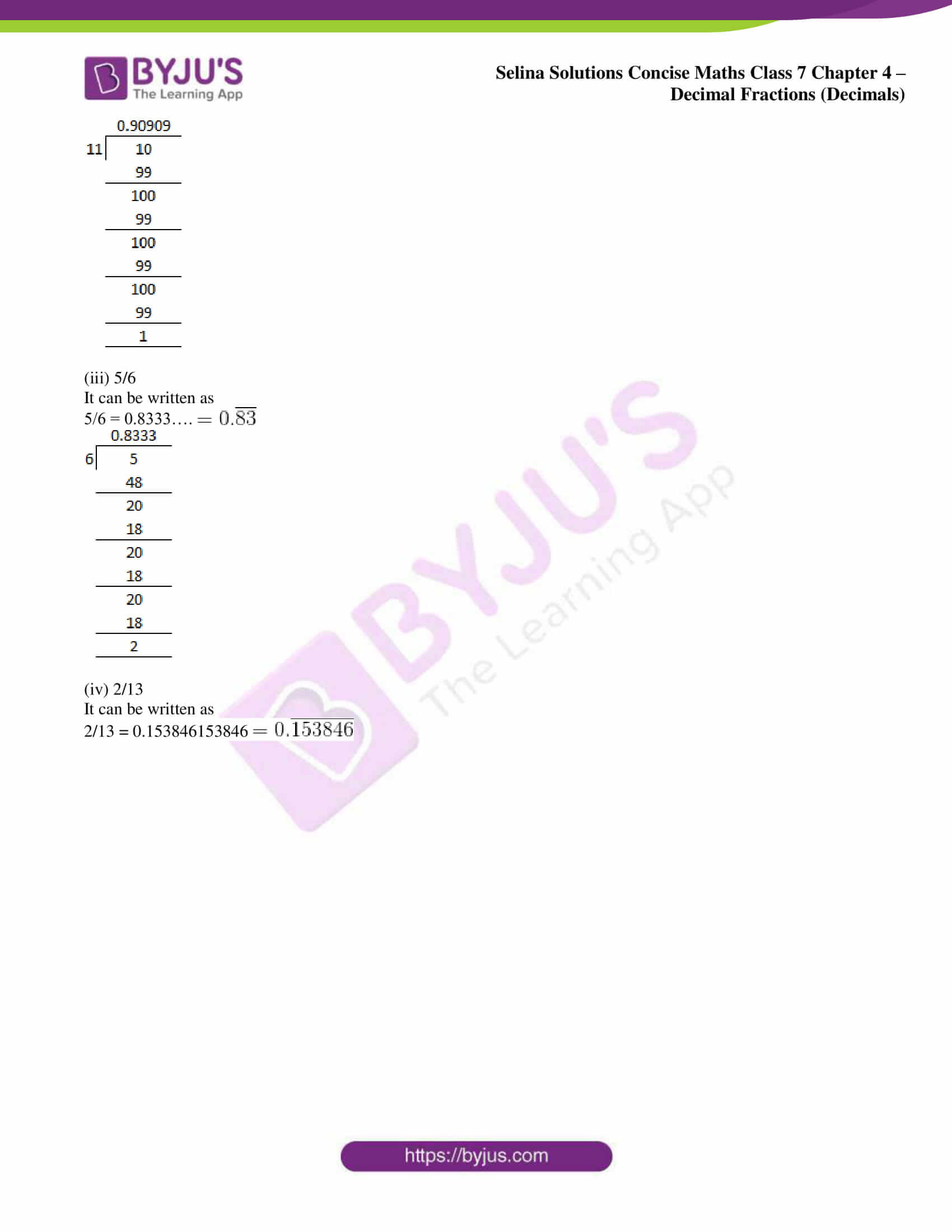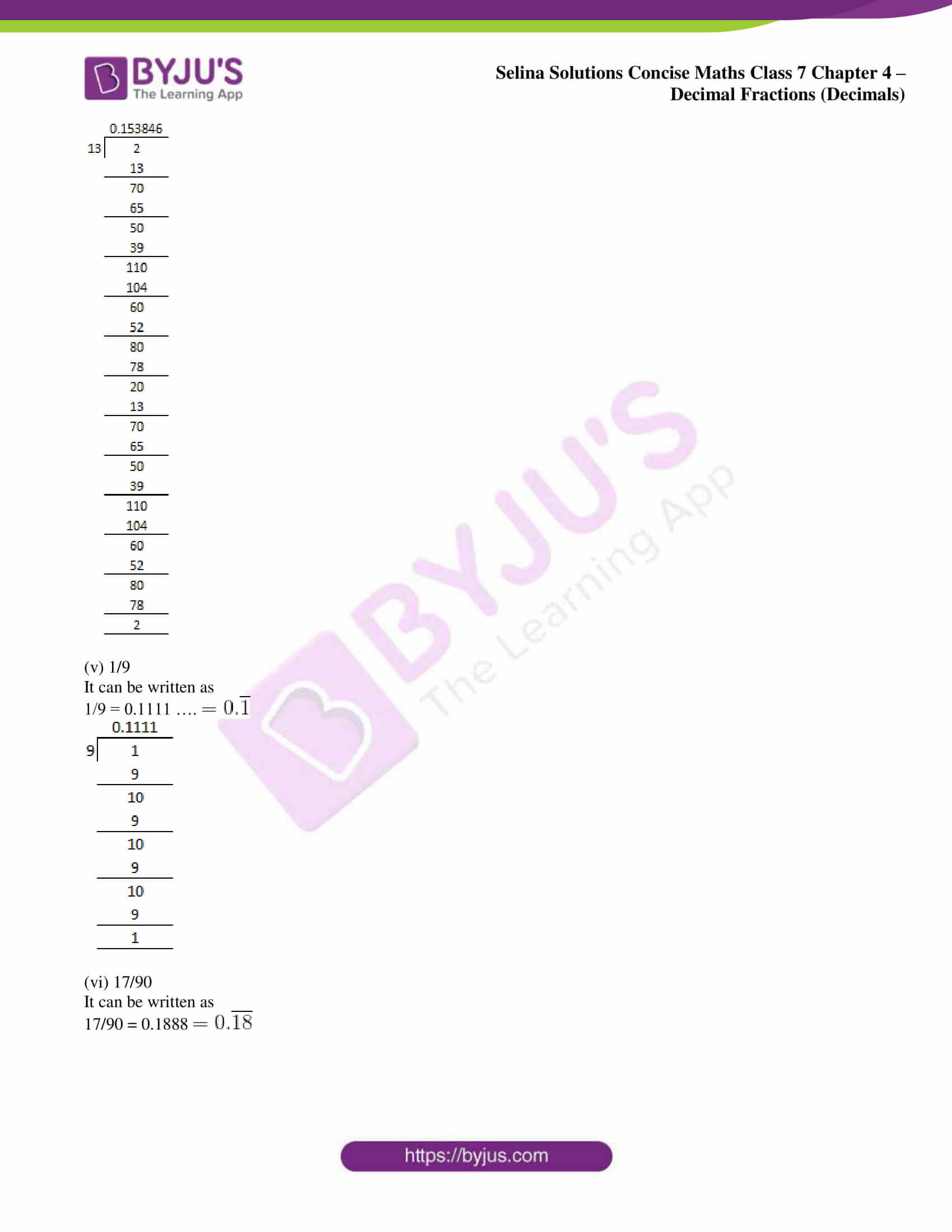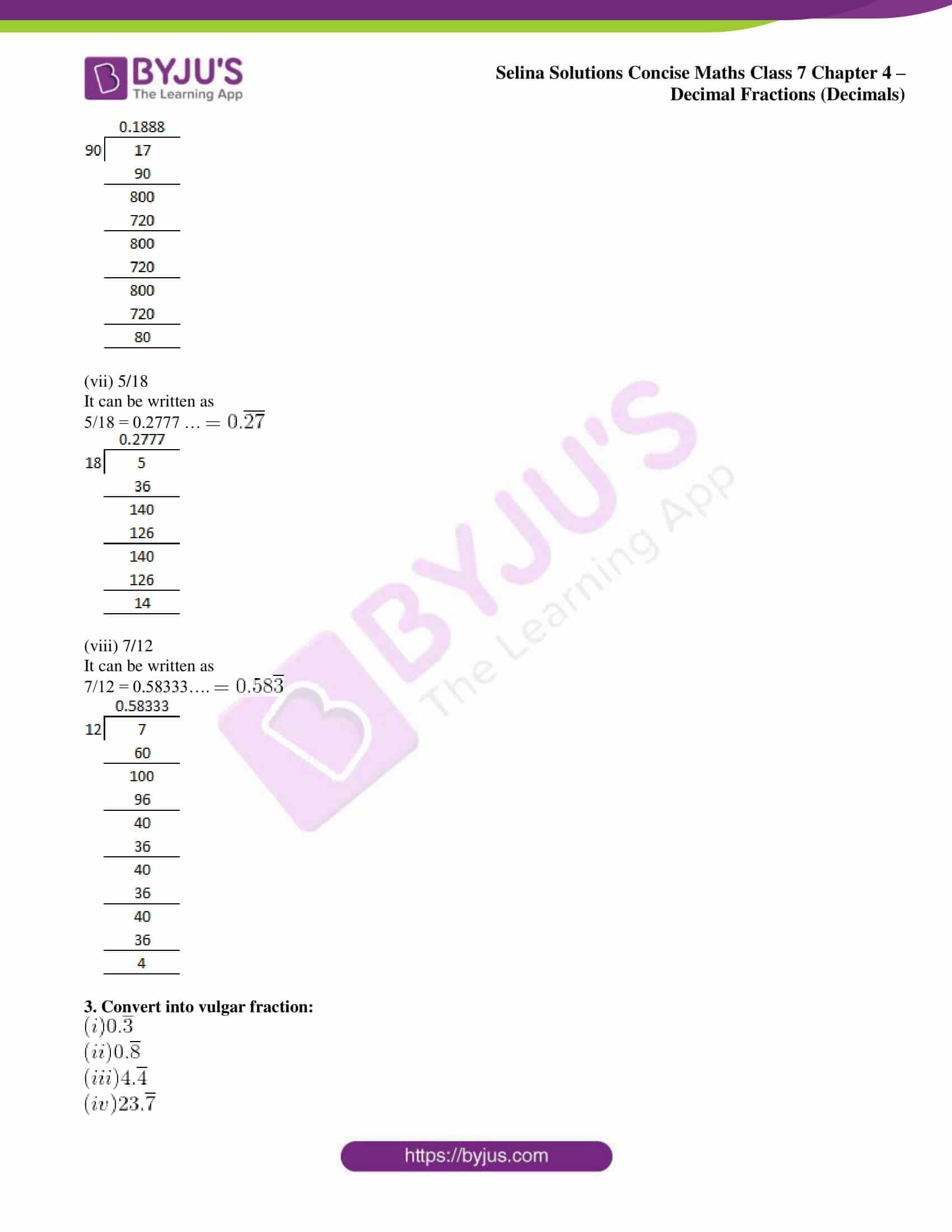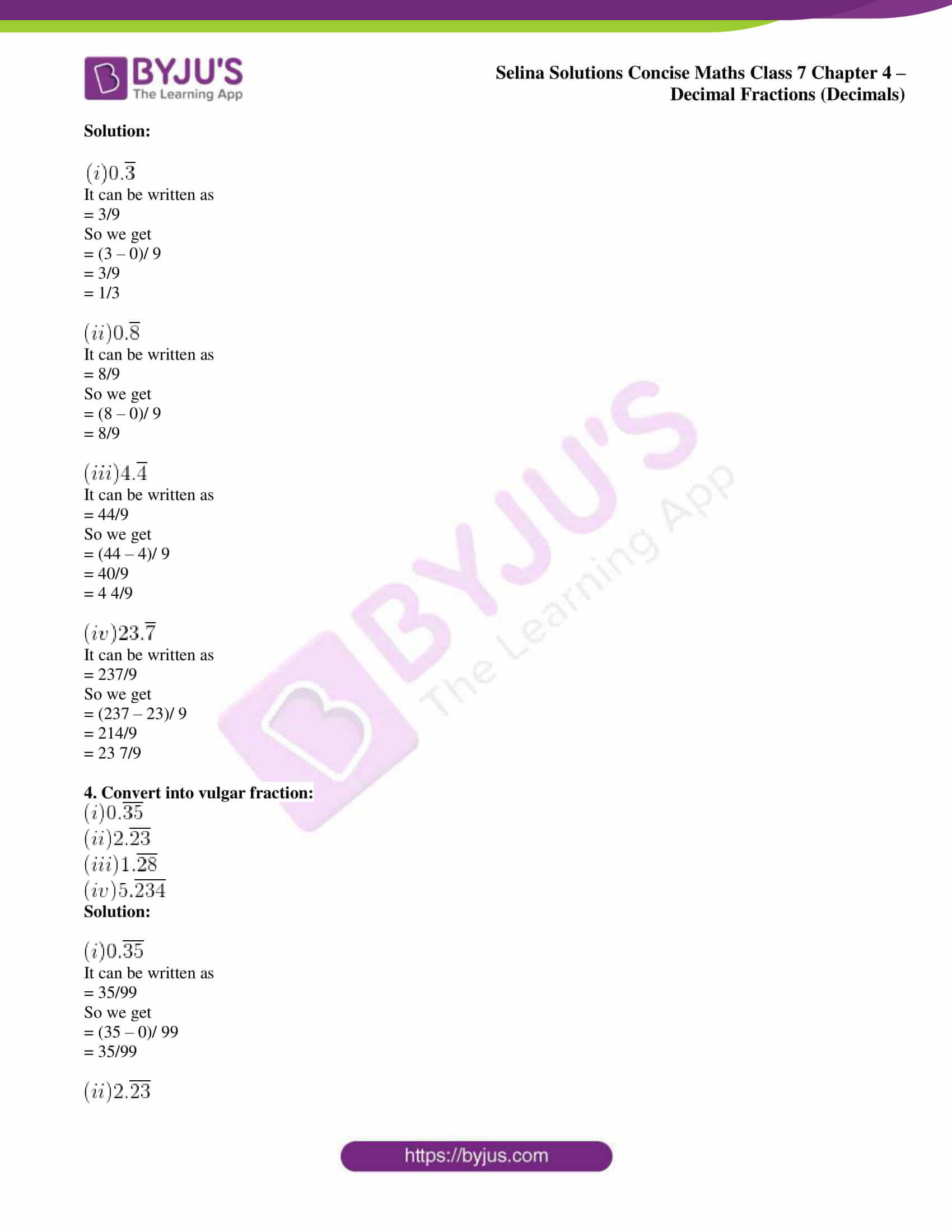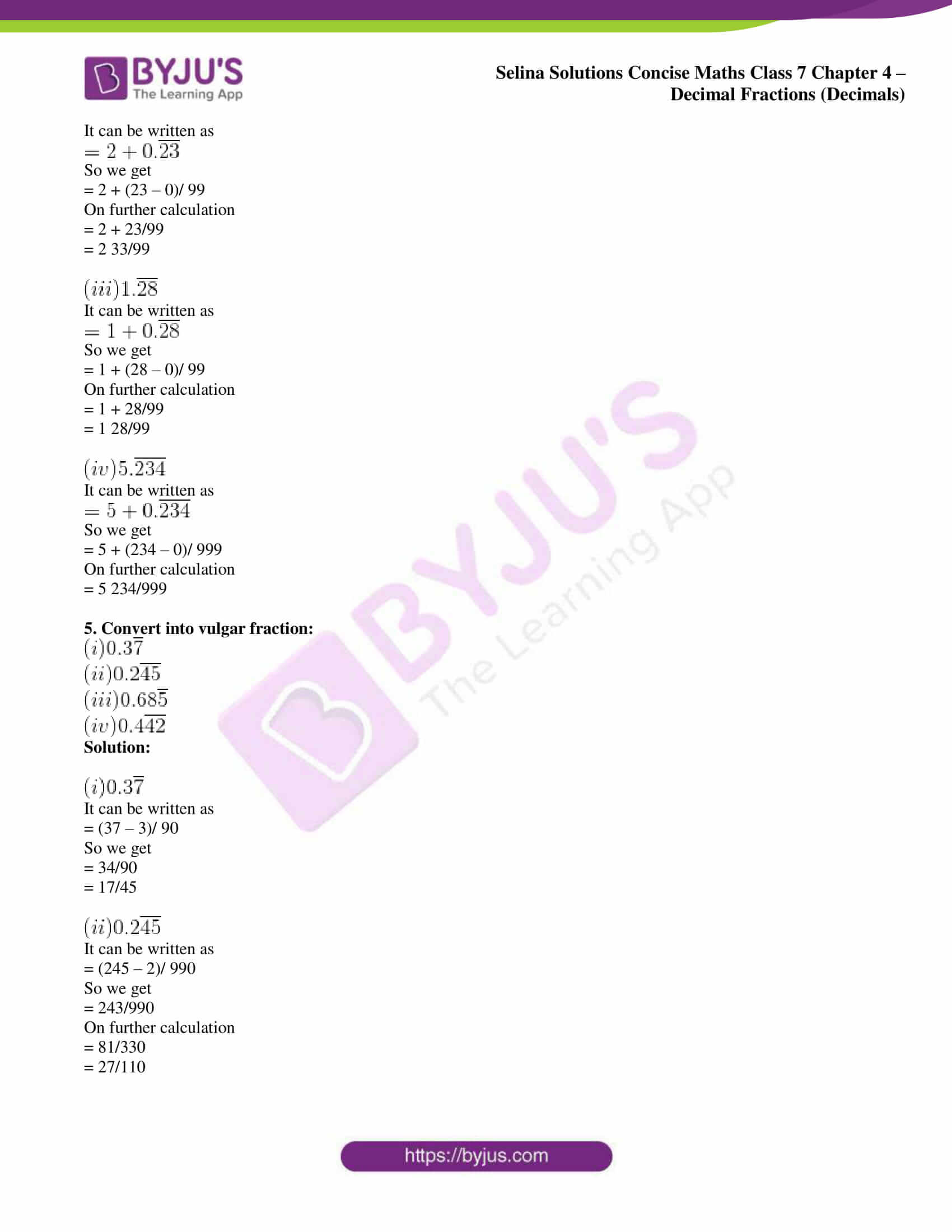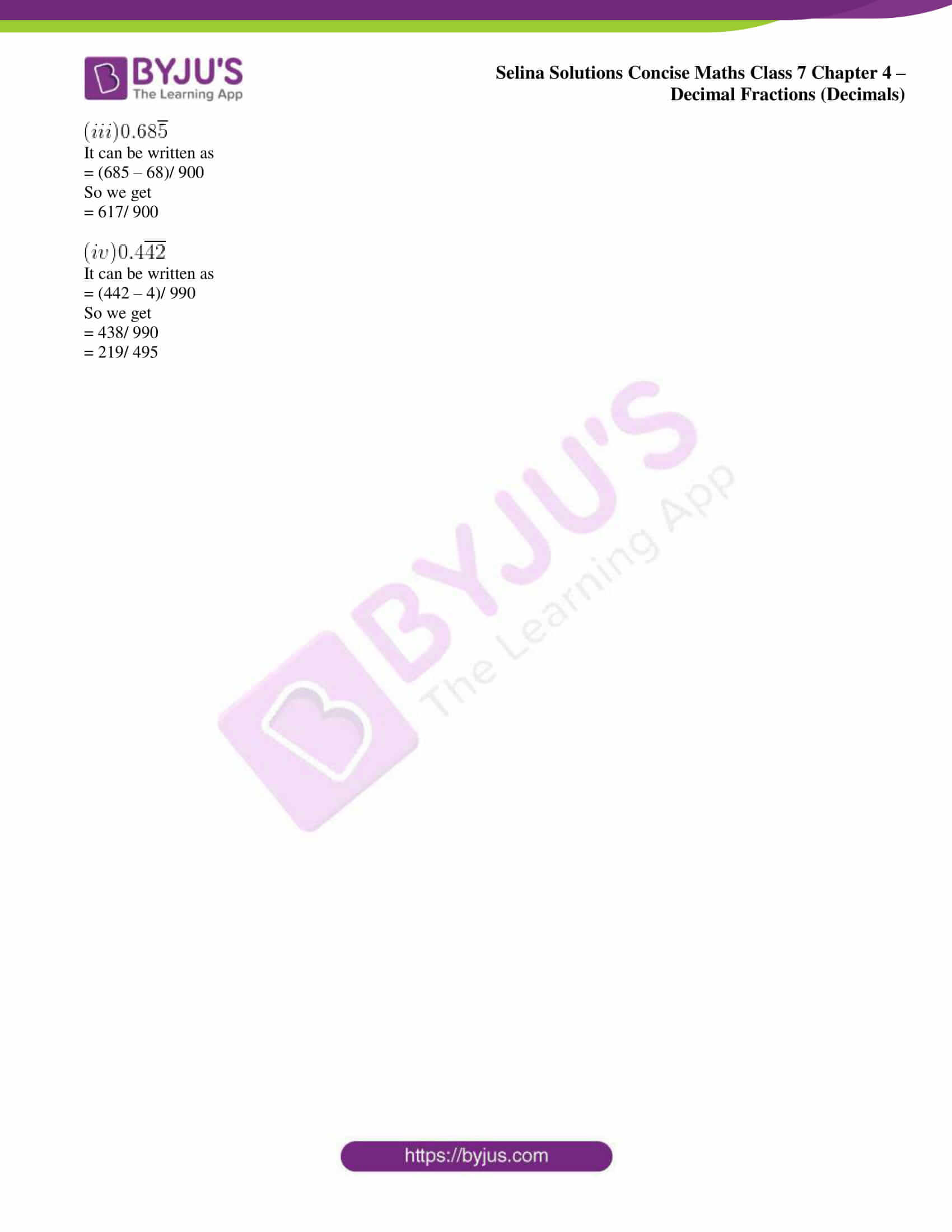### Access Selina Solutions Concise Maths Class 7 Chapter 4: Decimal Fractions (Decimals) Exercise 4D

1. Find whether the given division forms a terminating decimal or a non-terminating decimal:

(i) 3 ÷ 8

(ii) 8 ÷ 3

(iii) 6÷ 5

(iv) 5 ÷ 6

(v) 12.5 ÷ 4

(vi) 23 ÷ 0.7

(vii) 42 ÷ 9

(viii) 0.56÷ 0.11

Solution:

(i) 3 ÷ 8
We know that

3 ÷ 8 = 0.375

Therefore, it is terminating decimal.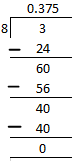(ii) 8 ÷ 3
We know that

8 ÷ 3 = 2.666

Therefore, it is a non-terminating decimal.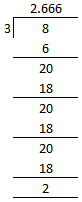(iii) 6 ÷ 5
We know that

6 ÷ 5 = 1.2

Therefore, it is terminating decimal.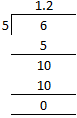(iv) 5 ÷ 6
We know that

5 ÷ 6 = 0.8333

Therefore, it is non-terminating decimal.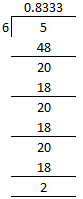(v) 12.5 ÷ 4
We know that

12.5 ÷ 4 = 3.125

Therefore, it is terminating decimal.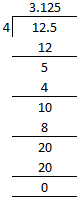(vi) 23 ÷ 0.7
Multiplying by 10 we get

230 ÷ 7 = 32.8571428

Therefore, it is non-terminating decimal.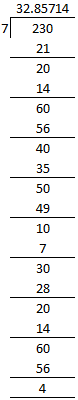(vii) 42 ÷ 9
We know that

42 ÷ 9 = 4.666

Therefore, it is non-terminating decimal.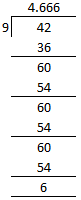(viii) 0.56 ÷ 0.11

Multiplying by 100

56 ÷ 11 = 5.0909

Therefore, it is non-terminating decimal.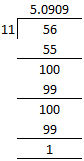2. Express as recurring decimals:

(i) 1 1/3

(ii) 10/11

(iii) 5/6

(iv) 2/13

(v) 1/9

(vi) 17/90

(vii) 5/18

(viii) 7/12

Solution:

(i) 1 1/3

It can be written as

1 1/3 = 4/3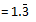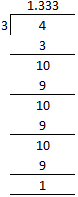(ii) 10/11

It can be written as

10/11 = 0.909090…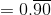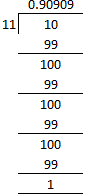(iii) 5/6

It can be written as

5/6 = 0.8333….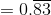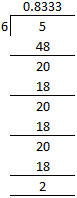(iv) 2/13

It can be written as

2/13 = 0.153846153846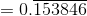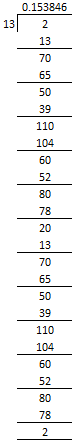(v) 1/9

It can be written as

1/9 = 0.1111 ….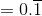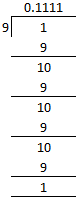(vi) 17/90

It can be written as

17/90 = 0.1888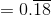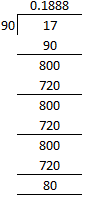(vii) 5/18

It can be written as

5/18 = 0.2777 …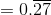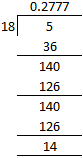(viii) 7/12

It can be written as

7/12 = 0.58333….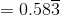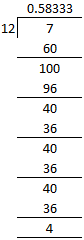3. Convert into vulgar fraction: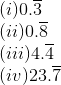Solution: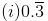It can be written as

= 3/9

So we get

= (3 – 0)/ 9

= 3/9

= 1/3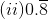It can be written as

= 8/9

So we get

= (8 – 0)/ 9

= 8/9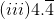It can be written as

= 44/9

So we get

= (44 – 4)/ 9

= 40/9

= 4 4/9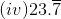It can be written as

= 237/9

So we get

= (237 – 23)/ 9

= 214/9

= 23 7/9

4. Convert into vulgar fraction: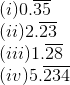Solution: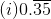It can be written as

= 35/99

So we get

= (35 – 0)/ 99

= 35/99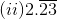It can be written as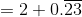So we get

= 2 + (23 – 0)/ 99

On further calculation

= 2 + 23/99

= 2 33/99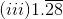It can be written as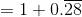So we get

= 1 + (28 – 0)/ 99

On further calculation

= 1 + 28/99

= 1 28/99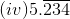It can be written as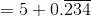So we get

= 5 + (234 – 0)/ 999

On further calculation

= 5 234/999

5. Convert into vulgar fraction: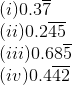Solution: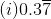It can be written as

= (37 – 3)/ 90

So we get

= 34/90

= 17/45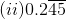It can be written as

= (245 – 2)/ 990

So we get

= 243/990

On further calculation

= 81/330

= 27/110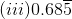It can be written as

= (685 – 68)/ 900

So we get

= 617/ 900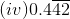It can be written as

= (442 – 4)/ 990

So we get

= 438/ 990

= 219/ 495